Divisibility Rules

What are the Divisibility Rules? - Math Divisibility Rules with Examples

The concept of division is tied with breaking something into many pieces. You share your sweets with your companions. You share bits of your favorite dishes at dinner with your friends and family. You begin with one amount and end up with little pieces.

To the extent that words are concerned, we will use three terms in this segment. In every division problem, you will have one number divided by another. The number you are breaking is known as the dividend. The solutions to your division problems are called quotients and remainders. Six divided by two gives you the rest of the three.
Just Like Fractions

This segment will move directly into the fractions region since fractions are fundamentally an alternate method for composing a division problem. When you move into further developed math, you may even work out division problems that resemble fractions.

You will discover that the fraction 1/4 has an indivisible incentive from one (1) partitioned by four (4). As you work with decimals, you will rapidly find that 1 divided by 4 is 0.25. The division is even imperative to rates. The decimal 0.25 is equivalent to saying 25 percent. One fourth of a pie is 25 % of the complete pie.

That example demonstrates to you that there are many approaches to state a similar thing in math. It resembles a language. A wide range of terms or words depict similar quantities, yet they all mean a similar thing. Blue, azul, bleu, blu, and blauw are largely extraordinary words that portray a similar shading of blue. You can move to start with one language then onto the next with some interpretation. You can move from fractions to decimals or rates with a little change.

Assume you're at a stationery shop and you have to discover which bargain is better by utilizing divisibility rules. Suppose 2 pencils cost Rs 6 and in another store, 4 pencils cost Rs 8. Which bargain is better? In the first case, each pencil costs Rs 3. In the second case, each pencil costs Rs 2. We realize that the shop with 4 pencils that cost Rs 8 is the better solution.

Long Division Method

Long division is an approach to take care of division problems with real numbers. These are division problems that you can't do in your mind.

How to write it down?

To start with, you need to record the problem in long division group. The normal division problem resembles this: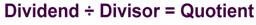To record this in long division place it would seem that this:How about we attempt a genuinely straightforward example: 187 ÷ 11 = ?

1. The first step is to put the problem into the long division group: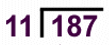2. The second step is to decide the smallest number to one side of the dividend, for this situation 187, which can be divided by 11. The number 1 is too little, so we take a gander at the first two numbers "18". Since 11 can fit into the number 18, so we can use it.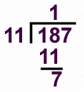In this way, we record how often 18 can be divided by 11. For this situation, the right response is 1. In the event that we attempted 2 which would be 22, which is greater than 18.

Next, we compose 11 underneath the 18 in light of the fact that 1 x 11 = 11. At that point, we subtract 11 from 18. This equals 7, which we record.

3. Since we have a digit 7 remaining, the problem isn't done. We presently move the 7 down from the end of the 187.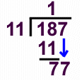4. In this way, we decide how often 11 will go into 77. That is actually multiple times. We record the 7 by the 1 in the right response zone. We record 77 underneath the 77 in light of the fact that 7 x 11 = 77.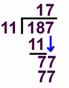5. Now we subtract 77 from 77. The right answer is zero. We have completed the problem. 187 ÷ 11 = 17.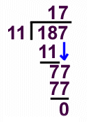A few tips for long division:

• • Write down a different table for the divisor before you begin the problem. For instance, if the divisor is 11 you record 11, 22, 33, 44, 55, 66, 77, 88, 99, and so forth. This can help you in avoiding mistakes.

• • Put a 0 in the left places of the remainder that you aren't utilizing. Ensure you keep every one of your numbers arranged. Composing perfectly and keeping the numbers arranged can truly help you with making fewer mistakes.

• • Double check the problem with verification. When you have your answer, do the problem backwards by multiplication to check whether your solution is correct or not.

• • The following are a couple of more instances of long division. Endeavor to work through these problems yourself to check whether you get similar outcomes.

•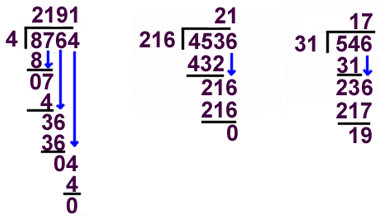Divisibility Tests

You have purchased 1235 chocolates and need to disperse them in your class. In what manner will you know with what number to partition them without really playing out the activity of division?

The procedure of whether the given number is exactly divisible by another number without really working out the process of division is known as the test of divisibility. Being exactly divisible implies that on division there is no leftover portion left. For understanding the divisibility rules for any number we have to know its divisibility with numbers like 2, 3, 4, 5, 9, 10 and 11.

Divisibility Rules

Divisibility Test for

On the off chance that the ones digit or unit's place digit of a number is either 0, 2, 4, 6 or 8 then the number is said to be divisible by 2. In different terms, on the off chance that the last digit of the number is even, at that point it is constantly divisible by 2. For instance, how about we take 24. The last digit is even. 4 is divisible by 2, hence the number 24 is divisible by 2.

Divisibility Test for

If the number formed by the last two digits is divisible by 4 then a number is said to be divisible by 4. For instance, how about we take 12343684. You don't have to stress over every one of the numbers, simply check the last 2 digits of the numbers. On the off chance that the last two digits i.e 84 are distinct by 4 then the number is divisible by 4.

Divisibility Tests for 3 and 9

A number is divisible by 3 if the sum of its digits is divisible by 3. We should view a few examples.
• 121: Here the sum of the digits is 4. Is 4 divisible by 3? No.
• 123: Here the sum of the digits is 6 and 6 is divisible by 3. So the number 123 is divisible by 3.
Similar rules are associated with the divisibility test of 9. A number is divisible by 9 if the sum of its digits is divisible by 9.

Divisibility Test for 5

A number is exactly divisible by 5 in the event that it has the digits 0 or 5 at ones place. The numbers like 15, 120, 205, 4405 and so forth are exactly divisible by 5 as these numbers have 0 or 5 in the ones place. How about we take a gander at an example.
In the numbers 21345650 and 459022, which one is divisible by 5? Out of both the numbers 21345650 and 459022, the first number i.e 21345650 is exactly divisible by 5 as the ones digit is 0.

Divisibility Test for 6

A number is divisible by 6 if that number is divisible by 2 and 3 both. For checking the divisibility of the number with 6 we need to apply divisibility tests that we perform for the divisors 2 and 3. We should take a gander at an example.
Check whether the 12581 is divisible by 6.

In the number 12581, the ones digit does not have any of the numbers like 0, 2, 4, 6, 8. So the number isn't divisible by 2. Henceforth the number isn't divisible by 6 on the grounds that for a number to be divisible by 6, it must be divisible by 2 and 3 both.

Divisibility Test for 8

The divisibility test for 8 is applied in the case of numbers with at least three digits. A number is divisible by 8 if the three digit number formed by the digits in ones, tens and the hundreds spots is divisible by 8. In the number 5864, we see that the number formed by the digits in ones, tens and hundreds spot is 864, which is divisible by 8, so the number is divisible by 8.

Divisibility Test for 10

If the units digit of a number is 0, then it is divisible by 10.

Divisibility Test for

Test 1:

Take the digits of the number backward, from right to left, multiplying them progressively by the digits 1, 3, 2, 6, 4, 5, repeating with this pattern of multipliers as long as necessary. On the off chance that the sum obtained is divisible by 7, then the number is divisible by 7.

Example: Is the number 1603 divisible by 7?
Solution: (3 × 1) + (0 × 3) + (6 × 2) + (1 × 6) = 21. This number is divisble by 7.

Test 2:

Double the last digit and subtract it from the remaining leading truncated number. In the event that the result is divisible by 7, then the original number is also divisible by 7.

Example: Is the number 1603 divisible by 7?
Solution: Applying the technique, 160 – 2× 6 = 154. This number is distinct by 7.

Divisibility Test by 11

If the difference of the sums of alternate digits of a number is 0 or a multiple of 11, then the number itself is divisible by 11. Let’s look at an example.

Ex: Check whether 19151 is divisible by 11 or not.
Solution: (1 + 1 + 1) – (9 + 5) = 3 – 14 = -11, which is divisible by 11, so 19151 is divisible by 11.

Solved Examples

Question: Which of the following numbers is divisible by 22?
Options:
(a) 4683
(b) 7106
(c) 3135
(d) 5682

Solution:

For a number to be divisible by 22, it must be divisible both by 2 and 11. Since A and C are odd numbers, they are not divisible by 22. In the case of D, (5 + 8) – (6 + 2) = 13 – 8 = 5, which is not a multiple of 11, so D is not divisible by 11, hence it is not divisible by 22. Since B is an even number, it is divisible by 2. Also, (7 + 0) – (1 + 6) = 7 – 7 = 0, hence 7106 is divisible by 11. So, B is divisible by 22. Hence the answer is B.